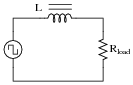# Mixed-Frequency Signals

## AC Electric Circuits

• #### Question 1

What is a harmonic frequency? If an oscillator circuit outputs a fundamental frequency of 12 kHz, calculate the frequencies of the following harmonics:

1st harmonic =
2nd harmonic =
3rd harmonic =
4th harmonic =
5th harmonic =
6th harmonic =
• #### Question 2

An interesting thing happens if we take the odd-numbered harmonics of a given frequency and add them together at certain diminishing ratios of the fundamental’s amplitude. For instance, consider the following harmonic series:

(1 volt at 100 Hz) + (1/3 volt at 300 Hz) + (1/5 volt at 500 Hz) + (1/7 volt at 700 Hz) + . . .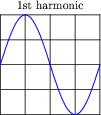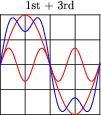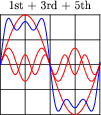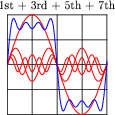Here is what the composite wave would look like if we added all odd-numbered harmonics up to the 13th together, following the same pattern of diminishing amplitudes: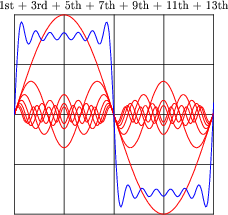If we take this progression even further, you can see that the sum of these harmonics begins to appear more like a square wave: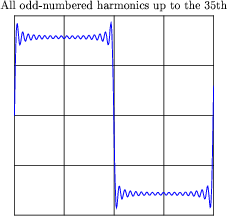This mathematical equivalence between a square wave and the weighted sum of all odd-numbered harmonics is very useful in analyzing AC circuits where square-wave signals are present. From the perspective of AC circuit analysis based on sinusoidal waveforms, how would you describe the way an AC circuit “views” a square wave?

• #### Question 3

In the early 1800’s, French mathematician Jean Fourier discovered an important principle of waves that allows us to more easily analyze non-sinusoidal signals in AC circuits. Describe the principle of the Fourier series, in your own words.

• #### Question 4

Identify the type of electronic instrument that displays the relative amplitudes of a range of signal frequencies on a graph, with amplitude on the vertical axis and frequency on the horizontal.

• #### Question 5

Suppose an amplifier circuit is connected to a sine-wave signal generator, and a spectrum analyzer used to measure both the input and the output signals of the amplifier: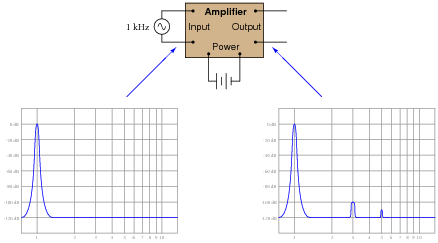Interpret the two graphical displays and explain why the output signal has more “peaks” than the input. What is this difference telling us about the amplifier’s performance?

• #### Question 6

What causes harmonics to form in the output of a transistor amplifier circuit, if the input waveform is perfectly sinusoidal (free from harmonics)? Be as specific as you can in your answer.

• #### Question 7

What causes harmonics to form in the output of a transistor oscillator circuit such as a Colpitts or a Hartley, which is designed to produce a sinusoidal signal? Be as specific as you can in your answer.

• #### Question 8

A clever way to produce sine waves is to pass the output of a square-wave oscillator through a low-pass filter circuit: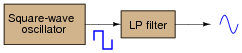Explain how this principle works, based on your knowledge of Fourier’s theorem.

• #### Question 9

What causes harmonics to form in AC electric power systems?

• #### Question 10

Explain how the following power-line harmonic analyzer circuit works:Harmonic # L# value C# value 1st 20 to 22 H 0.33 μF 2nd 11 to 12 H 0.15 μF 3rd 5 to 6 H 0.15 μF 4th 1.5 to 2.5 H 0.22 μF 5th 1 to 1.5 H 0.27 μF

• #### Question 11

What is a harmonic frequency? If a particular electronic system (such as an AC power system) has a fundamental frequency of 60 Hz, calculate the frequencies of the following harmonics:

1st harmonic =
2nd harmonic =
3rd harmonic =
4th harmonic =
5th harmonic =
6th harmonic =
• #### Question 12

An octave is a type of harmonic frequency. Suppose an electronic circuit operates at a fundamental frequency of 1 kHz. Calculate the frequencies of the following octaves:

1 octave greater than the fundamental =
2 octaves greater than the fundamental =
3 octaves greater than the fundamental =
4 octaves greater than the fundamental =
5 octaves greater than the fundamental =
6 octaves greater than the fundamental =
• #### Question 13

The Fourier series for a square wave is as follows:

 vsquare = 4 π Vm ( sinωt + 1 3 sin3 ωt + 1 5 sin5 ωt + 1 7 sin7 ωt … + 1 n + sinn ωt )

Where,

Vm = Peak amplitude of square wave

ω = Angular velocity of square wave (equal to 2 πf, where f is the fundamental frequency)

n = An odd integer

Electrically, we might represent a square-wave voltage source as a circle with a square-wave symbol inside, like this: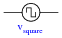Knowing the Fourier series of this voltage, however, allows us to represent the same voltage source as a set of series-connected voltage sources, each with its own (sinusoidal) frequency. Draw the equivalent schematic for a 10 volt (peak), 200 Hz square-wave source in this manner showing only the first four harmonics, labeling each sinusoidal voltage source with its own RMS voltage value and frequency:

Hint: ω = 2 πf

• #### Question 14

Suppose a non-sinusoidal voltage source is represented by the following Fourier series:

 v(t) = 23.2 + 30 sin(377t) + 15.5 sin(1131t + 90) + 2.7 sin(1508t - 40)

Electrically, we might represent this non-sinusoidal voltage source as a circle, like this: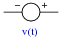Knowing the Fourier series of this voltage, however, allows us to represent the same voltage source as a set of series-connected voltage sources, each with its own (sinusoidal) frequency. Draw the equivalent schematic in this manner, labeling each voltage source with its RMS voltage value, frequency (in Hz), and phase angle:

Hint: ω = 2 πf

• #### Question 15

Calculate the power dissipated by a 25 Ω resistor, when powered by a square-wave with a symmetrical amplitude of 100 volts and a frequency of 2 kHz: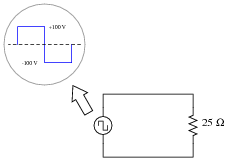• #### Question 16

Calculate the power dissipated by a 25 Ω resistor, when powered by a square-wave with a symmetrical amplitude of 100 volts and a frequency of 2 kHz, through a 0.22 μF capacitor: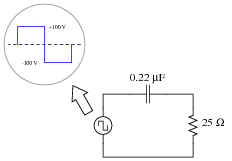No, I’m not asking you to calculate an infinite number of terms in the Fourier series - that would be cruel and unusual. Just calculate the power dissipated in the resistor by the 1st, 3rd, 5th, and 7th harmonics only.

• #### Question 17

Ideally, a sinusoidal oscillator will output a signal consisting of a single (fundamental) frequency, with no harmonics. Realistically, though, sine-wave oscillators always exhibit some degree of distortion, and are therefore never completely harmonic-free.

Describe what the display of a spectrum analyzer would look like when connected to the output of a perfect sinusoidal oscillator. Then, describe what the same instrument’s display would look like if the oscillator exhibited substantial distortion.

• #### Question 18

An electronics technician connects the input of a spectrum analyzer to the secondary winding of an AC power transformer, plugged into a power receptacle. He sets the spectrum analyzer to show 60 Hz as the fundamental frequency, expecting to see the following display: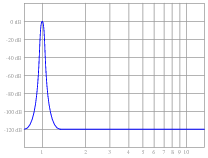Instead, however, the spectrum analyzer shows more than just a single peak at the fundamental: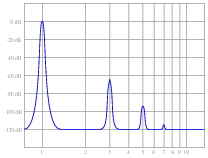Explain what this pattern means, in practical terms. Why is this power system’s harmonic signature different from what the technician expected to see?

• #### Question 19

Ideally, an amplifier circuit increases the amplitude of a signal without altering the signal’s wave-shape in the least. Realistically, though, amplifiers always exhibit some degree of distortion.

Describe how harmonic analysis - either with a spectrum analyzer or some other piece of test equipment capable of measuring harmonics in a signal - is used to quantify the distortion of an amplifier circuit.

• #### Question 20

Under certain conditions, harmonics may be produced in AC power systems by inductors and transformers. How is this possible, as these devices are normally considered to be linear?

• #### Question 21

Identify some ways in which harmonics may be mitigated in AC power systems, since they tend to cause trouble for a variety of electrical components.

• #### Question 22

If both these circuits are energized by an AC sine-wave source providing a perfectly undistorted signal, the resulting output waveforms will differ in phase and possibly in amplitude, but not in shape: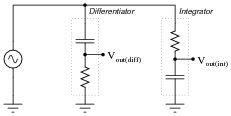If, however, the excitation voltage is slightly distorted, one of the outputs will be more sinusoidal than the other. Explain whether it is the differentiator or the integrator that produces the signal most resembling a pure sine wave, and why.

Hint: I recommend building this circuit and powering it with a triangle wave, to simulate a mildly distorted sine wave.

• #### Question 23

Note the effect of adding the second harmonic of a waveform to the fundamental, and compare that effect with adding the third harmonic of a waveform to the fundamental: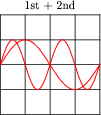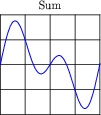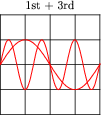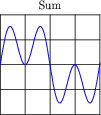Now compare the sums of a fundamental with its fourth harmonic, versus with its fifth harmonic: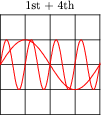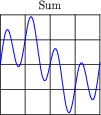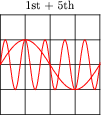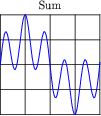And again for the 1st 6th, versus the 1st 7th harmonics: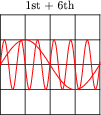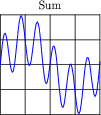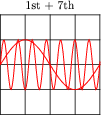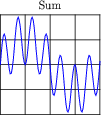Examine these sets of harmonic sums, and indicate the trend you see with regard to harmonic number and symmetry of the final (Sum) waveforms. Specifically, how does the addition of an even harmonic compare to the addition of an odd harmonic, in terms of final waveshape?

• #### Question 24

When technicians and engineers consider harmonics in AC power systems, they usually only consider odd-numbered harmonic frequencies. Explain why this is.

• #### Question 25

By visual inspection, determine which of the following waveforms contain even-numbered harmonics:Note that only one cycle is shown for each waveform. Remember that we’re dealing with continuous waveforms, endlessly repeating, and not single cycles as you see here.

• #### Question 26

By visual inspection, determine which of the following waveforms contain even-numbered harmonics: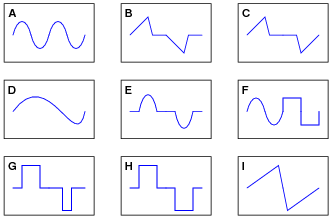Note that only one cycle is shown for each waveform. Remember that we’re dealing with continuous waveforms, endlessly repeating, and not single cycles as you see here.

• #### Question 27

A crude measurement circuit for harmonic content of a signal uses a notch filter tuned to the fundamental frequency of the signal being measured. Examine the following circuit and then explain how you think it would work: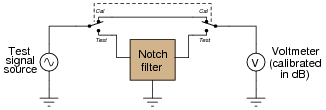• #### Question 28

Radio communication functions on the general principle of high-frequency AC power being modulated by low-frequency data. Two common forms of modulation are Amplitude Modulation (AM) and Frequency Modulation (FM). In both cases, the modulation of a high frequency waveform by a lower-frequency waveform produces something called sidebands.

Describe what “sidebands” are, to the best of your ability.

• #### Question 29

The following circuit is a simple mixer circuit, combining three AC voltage signals into one, to be measured by an oscilloscope: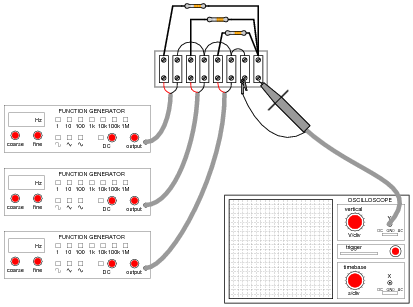Draw a schematic diagram of this circuit, to make it easier to analyze.

Is it possible to filter the three constituent input signals from each other in the resulting output signal, or are they irrevocably affected by one another when they “mix” together in this resistor network? How does the superposition principle relate to the operation of a mixer circuit like this?

What if the mixing circuit contains capacitors and inductors rather than resistors? Does the same principle apply? Why or why not?

• #### Question 30

What is a musical chord? If viewed on an oscilloscope, what would the signal for a chord look like?